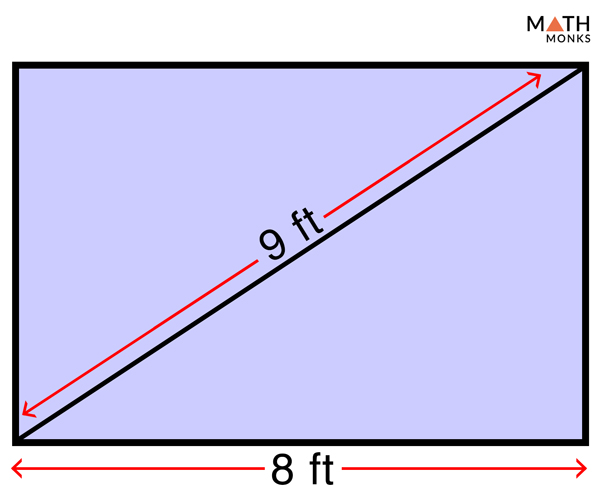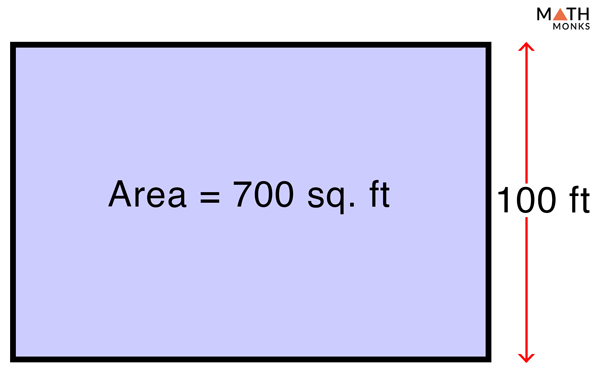# Perimeter of Rectangle

The perimeter of any two-dimensional geometric shape is the distance covered around its edge. In this article, we will learn how to find the perimeter of a rectangle with formulas and examples.

## What is the Perimeter of a Rectangle

The perimeter of a rectangle is the total distance covered around the edge of the rectangle.

Since a rectangle is a four-sided shape, its perimeter is also defined as the sum of its four sides.

Perimeter is the total measure of length or distance. Expression of the perimeter is in linear units. So the unit of the perimeter of a rectangle is yards, feet, inches, or meters, or any other linear unit.

## Formula

The formula to find the perimeter of a rectangle is given below:

### Derivation

Since the perimeter of a quadrilateral (a geometric shape with 4 sides) is the sum of all the sides, therefore, the perimeter (P) is,

P = sum of all the four sides = w + w + l + l = 2(w + l), where w = width, and l = length.

Thus we get the standard formula of Perimeter of a rectangle (P) = 2(w + l)

### How to Find the Perimeter of a Rectangle

The basic steps for calculating the perimeter of a rectangle given its length (l) and width (w) are as follows:

• Write down the formula of Perimeter of a rectangle 2(w + l).
• Substitute the values of length (l) and width (w) in the formula.
• Write down the answer in linear units.

Let us solve some examples to understand the concept better.

## Solved ExamplesFind the perimeter of a rectangular garden 10 m long and 6 m wide.

Solution:

As we know,
P = 2(w + l), here w = 6 m, l = 10 m
= 2 x (10 + 6)
= 32 m.

How much lace will John need to decorate a bedsheet 150 inches long and 95 inches wide?

Solution:

The length of the lace is equal the perimeter (P) of the bed sheet.
As we know,
P = 2(w + l), here, w = 95 inches, l = 150 inches
= 2 x (95 + 150)
= 490 inches
So, John needs a lace of 490 inches to decorate the bedsheet.

Finding the perimeter of a rectangle when the DIAGONAL and any ONE SIDE are knownFind the perimeter of a rectangle with a diagonal of 9 feet and a length of 8 feet.

Solution:

Here we will use the formula P = 2l + 2√(d2 – l2) as d = √(w2 + l2) where d = diagonal, l = length
As we know,
P = 2l + 2√(d2 – l2), where d = 9 ft, and l = 8 ft
= 2 x 8 + 2 x √(92 – 82)
≈ 24.25 ft

Find the perimeter of a rectangle 16 inches wide with a diagonal of 32 inches.

Solution:

Here we will use the formula P = 2w + 2√(d2 – w2) as d = √(w2 + l2) where d = diagonal, w = width
As we know,
P  = 2w + 2√(d2 – w2), where w = 16 inches, d = 32 inches
= 2 x 16 + 2√(322 – 162)
≈ 87.42 inches

Finding the perimeter of a rectangle when AREA and any ONE SIDE are knownFind the perimeter of a rectangular swimming pool whose area is 700 sq. ft and is 100 ft wide.

Solution:

Here we will use the formula P = 2w + 2A/w to find the perimeter (P) as A = w × l and P = 2(w + l), Where w = width, l = length, A = area.
As we know,
P = 2w + 2A/w, here  w = 100 ft, and A = 700 sq. ft.
= 2 × 100 + 2 × (700/100)
= 214 ft.

Find the perimeter of a rectangle whose area is 3750 sq. cm and length is 75 cm.

Solution:

Here we will use the formula P = 2l + 2A/l to find the perimeter (P) as A = w × l and P = 2(w + l),
Where w = width, l = length, A = area.
As we know,
P = 2l + 2A/l, here l = 75 cm, and A = 3750 sq. cm
= 2 × 75 + 2 × (3750/75)
= 250 cm

Finding the measure of any one side when PERIMETER and any ONE SIDE are known

Find the length of the park if the length of a park is twice its width, and the perimeter is 390 yards.

Solution:

Here we will use the formula l = P/2 – w, which we get from the formula P = 2(w + l), where l = length, P = perimeter, w = width.
As we know,
l = P/2 – w, here l = 2 x w, so w = l/2, and P = 390
or, P = 2{(l/2) + 2w} (substituting the value of w as l/2 in the equation)
or, 3l = P,
or,  l = P/3
= 390/3 (since P = 390)
= 130 yards.
The length of the park is 130 yards.